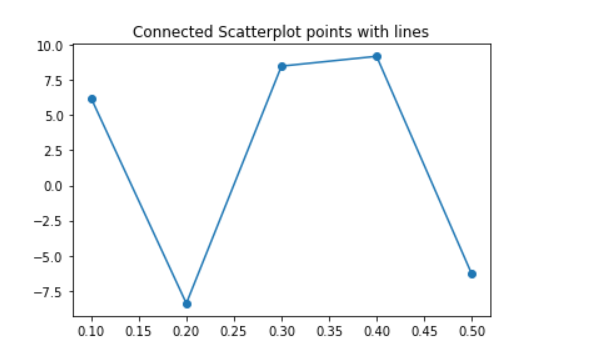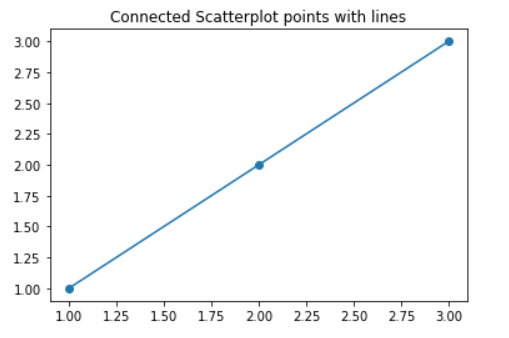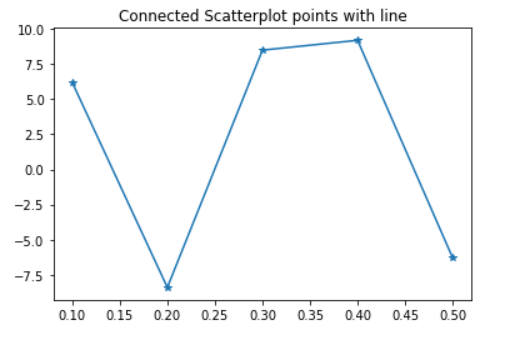GFG App
Open AppBrowser
Continue

# How to Connect Scatterplot Points With Line in Matplotlib?

Prerequisite: Scatterplot using Seaborn in Python

Scatterplot can be used with several semantic groupings which can help to understand well in a graph. They can plot two-dimensional graphics that can be enhanced by mapping up to three additional variables while using the semantics of hue, size, and style parameters. And matplotlib is very efficient for making 2D plots from data in arrays. In this article, we are going to see how to connect scatter plot points with lines in matplotlib.

Approach:

• Import module.
• Determined X and Y coordinate for plot scatter plot points.
• Plot scatterplot.
• Plot matplotlib.pyplot with the same X and Y coordinate.

Below is the implementation:

Example 1:

## Python3

 `# import module ` `import` `numpy as np ` `import` `matplotlib.pyplot as plt ` ` `  `# initialize x and y coordinates ` `x ``=` `[``0.1``, ``0.2``, ``0.3``, ``0.4``, ``0.5``] ` `y ``=` `[``6.2``, ``-``8.4``, ``8.5``, ``9.2``, ``-``6.3``] ` ` `  `# set the title of a plot ` `plt.title(``"Connected Scatterplot points with lines"``) ` ` `  `# plot scatter plot with x and y data ` `plt.scatter(x, y) ` ` `  `# plot with x and y data ` `plt.plot(x, y) `

Output:Example 2:

## Python3

 `# import module ` `import` `numpy as np ` `import` `matplotlib.pyplot as plt ` ` `  `# initialize x and y coordinates ` `x ``=` `[``1``, ``2``, ``3``] ` `y ``=` `[``1``, ``2``, ``3``] ` ` `  `# set the title of a plot ` `plt.title(``"Connected Scatterplot points with lines"``) ` ` `  `# plotting scatter and pyplot ` `plt.scatter(x, y) ` `plt.plot(x, y) `

Output:Example 3:

We can also connect scatter plot points with lines without using seaborn.scatterplot. We will use only pyplot to connect the scatter points with lines.

## Python3

 `# import module ` `import` `numpy as np ` `import` `matplotlib.pyplot as plt ` ` `  `# initialize x and y coordinates ` `x ``=` `[``0.1``, ``0.2``, ``0.3``, ``0.4``, ``0.5``] ` `y ``=` `[``6.2``, ``-``8.4``, ``8.5``, ``9.2``, ``-``6.3``] ` ` `  `plt.title(``"Connected Scatterplot points with line"``) ` `plt.plot(x, y, marker``=``"*"``) ` `plt.show() `

Output:My Personal Notes arrow_drop_up